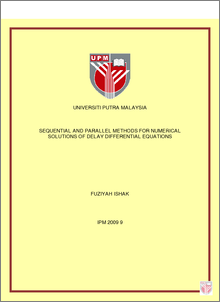Simple Search:

Sequential and Parallel Methods for Numerical Solutions of Delay Differential Equations

Citation

Ishak, Fuziyah (2009) Sequential and Parallel Methods for Numerical Solutions of Delay Differential Equations. PhD thesis, Universiti Putra Malaysia.

Abstract / Synopsis

This thesis describes the development of sequential and parallel methods for solving delay differential equations. A new sequential code for the numerical solution of delay differential equations is considered. The variable order variable stepsize formulae based on the Adams-Bashforth-Moulton methods are represented in divided difference form. Derivative discontinuities are detected by local error estimate at the grid points. Large magnitude of the local error estimate indicates the presence of derivative discontinuity. Stepsize is then reduced and eventually the discontinuity point is included in the grid. The formulae representation proves to be efficient when compared with the existing method in modified divided difference form. We also consider the development of two-point block methods on sequential and parallel computers. Formulae for three two-point block methods for solving delay differential equations are derived. The implicit block methods are implemented using variable stepsize variable order technique. The formulae for two-point diagonally and triangu larly implicit block methods using predictor-corrector application are represented in divided difference form. Meanwhile, the predictor-corrector formulae for two-point fully implicit block method are calculated beforehand and stored at the beginning of the code. All of the block methods rely on the local error estimates to detect derivative discontinuities. In all of the developed methods, regions of absolute stability are presented and compared. Comparison among the developed methods indicates that all of the methods achieve the desired accuracy. Block methods are efficient when compared with the sequential non-block method as the total steps taken can be reduced. The new block methods are then used for the parallel implementation in solving large system of delay differential equations. The parallel programs using Message Passing Interface are run on Sun Fire V1280 using two processors. Numerical results indicate that parallel implementation increases the performance of the block methods.Preview
PDF
ABS_----__IPM_2009_9.pdfView Item